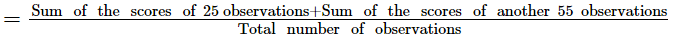# The mean score of 25 observations is 80 and the mean score of another 55 observations is 60.

Question:

The mean score of 25 observations is 80 and the mean score of another 55 observations is 60. Determine the mean score of the whole set of observations.

Solution:

Mean score of 25 observations $=80$

Sum of the scores of 25 observations $=80 \times 25=2000$

Mean score of another 55 observations $=60$

Sum of the scores of another 55 observations $=60 \times 55=3300$

Mean score of the whole set of observations$=\frac{2000+3300}{80}$

$=\frac{5300}{80}$

$=66.25$

Therefore, the mean score of the whole set of observations is 66.25.